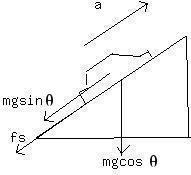# Question# A sports car is accelerating up a hill that rises 18.0^\circ above the horizontal. The coefficient of static friction between the wheels and the road

Other
ANSWEREDA sports car is accelerating up a hill that rises $$\displaystyle{18.0}^{\circ}$$ above the horizontal. The coefficient of static friction between the wheels and the road is $$\displaystyle\mu{s}={0.77}$$.It is the static frictional force that propels the car forward.
(a) What is the magnitude of the maximumacceleration that the car can have?
(b) What is the magnitude of the maximum acceleration if the car isbeing driven down the hill?2021-04-16
First we have draw the free body diagramThe angle between horizontal and inclined plane is $$\displaystyle\theta={18}^{\circ}$$
a) From the diagram we can write
$$\displaystyle{m}{g}{\sin{\theta}}+{f}_{{s}}={m}{a}$$
$$\displaystyle{m}{g}{\sin{\theta}}+\mu_{{s}}{m}{g}{\cos{\theta}}={m}{a}$$
Therefore the magnitude od acceleration
$$\displaystyle{a}={g{{\left({\sin{\theta}}+\mu_{{s}}{m}{g}{\cos{\theta}}={m}{a}\right.}}}$$
b) For this in the diagram the direction offriction reversed.
Therefore we can write
$$\displaystyle{m}{g}{\sin{\theta}}-\mu_{{s}}{m}{g}{\cos{\theta}}={m}{a}$$
Therefore the magnitude of acceleration
$$\displaystyle{a}={g{{\left({\sin{\theta}}-\mu_{{s}}{\cos{\theta}}={m}{a}\right.}}}$$
substitute the given values.